SBOS947A July   2019  – June 2020

PRODUCTION DATA.

1. Features
2. Applications
3. Description
1.     Device Images
4. Revision History
5. Pin Configuration and Functions
6. Specifications
7. Detailed Description
1. 7.1 Overview
2. 7.2 Functional Block Diagram
3. 7.3 Feature Description
4. 7.4 Device Functional Modes
8. Application and Implementation
1. 8.1 Application Information
2. 8.2 Typical Applications
9. Power Supply Recommendations
10. 10Layout
11. 11Device and Documentation Support
1. 11.1 Device Support
1. 11.1.1 Development Support
2. 11.2 Documentation Support
3. 11.3 Receiving Notification of Documentation Updates
4. 11.4 Support Resources
5. 11.5 Trademarks
6. 11.6 Electrostatic Discharge Caution
7. 11.7 Glossary
12. 12Mechanical, Packaging, and Orderable Information

• D|8
• DSG|8
• DSG|8

### Table 1. Typical Characteristic Graphs

DESCRIPTION FIGURE
Offset Voltage Distribution Figure 1
Offset Voltage Drift (-40°C to +125C°C) Figure 2
Input Bias Current Distribution Figure 3
Input Offset Current Distribution Figure 4
Offset Voltage vs Common-Mode Voltage Figure 5
Offset Voltage vs Supply Voltage Figure 6
Open-Loop Gain and Phase vs Frequency Figure 7
Closed-Loop Gain vs Frequency Figure 8
Input Bias Current and Offset Current vs Temperature Figure 9
Output Voltage Swing vs Output Current (Sourcing) Figure 10
Output Voltage Swing vs Output Current (Sinking) Figure 11
CMRR and PSRR vs Frequency Figure 12
CMRR vs Temperature Figure 13
PSRR vs Temperature Figure 14
0.1-Hz to 10-Hz Voltage Noise Figure 15
Input Voltage Noise Spectral Density vs Frequency Figure 16
THD+N vs Frequency Figure 17
THD+N vs Output Amplitude Figure 18
Quiescent Current vs Supply Voltage Figure 19
Quiescent Current vs Temperature Figure 20
Open-Loop Gain vs Temperature (10 kΩ) Figure 21
Open-Loop Gain vs Temperature (2 kΩ) Figure 22
Open-Loop Output Impedance vs Frequency Figure 23
Small-Signal Overshoot vs Capacitive Load (Gain = –1, 10-mV step) Figure 24
Small-Signal Overshoot vs Capacitive Load (Gain = 1, 10-mV step) Figure 25
No Phase Reversal Figure 26
Positive Overload Recovery Figure 27
Negative Overload Recovery Figure 28
Small-Signal Step Response (Gain = 1, 10-mV step) Figure 29
Small-Signal Step Response (Gain = –1, 10-mV step) Figure 30
Large-Signal Step Response (Gain = 1, 10-V step) Figure 31
Large-Signal Step Response (Gain = –1, 10-V step) Figure 32
Phase Margin vs Capacitive Load Figure 33
Settling Time (1-V Step, 0.1% Settling) Figure 34
Short Circuit Current vs Temperature Figure 35
Maximum Output Voltage vs Frequency Figure 36
EMIRR vs Frequency Figure 37
Channel Separation Figure 38
at TA = 25°C, VS = ±12 V, VCM = VS / 2, RL = 10 kΩ (unless otherwise noted)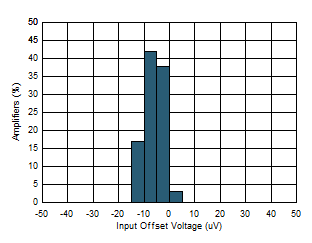Figure 1. Offset Voltage Distribution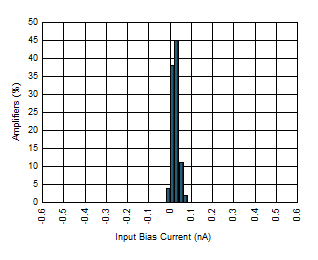Figure 3. Input Bias Current Distribution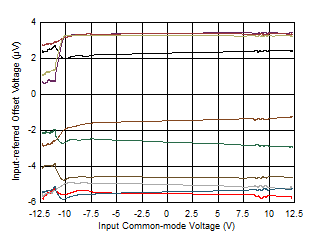Figure 5. Offset Voltage vs Common-Mode Voltage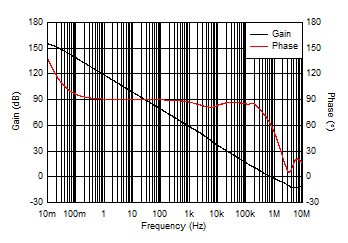Figure 7. Open-Loop Gain and Phase vs Frequency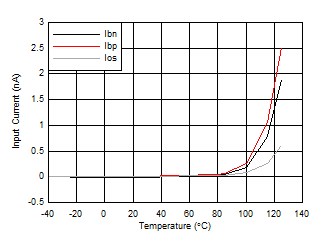Figure 9. Input Bias Current and Offset Current vs Temperature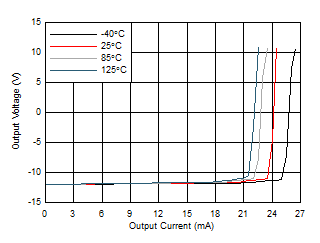Figure 11. Output Voltage Swing vs Output Current (Sinking)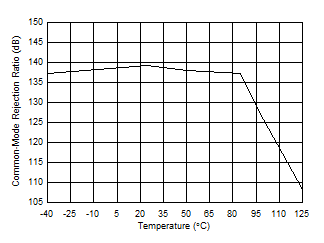Figure 13. CMRR vs Temperature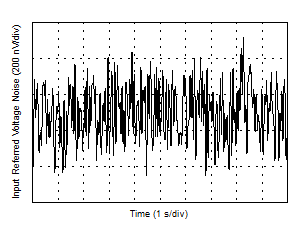Figure 15. 0.1-Hz to 10-Hz Voltage Noise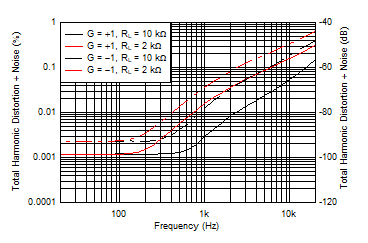Figure 17. THD+N vs Frequency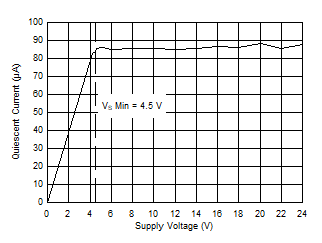Figure 19. Quiescent Current vs Supply Voltage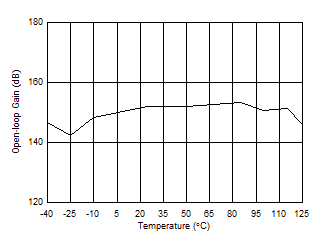Figure 21. Open-Loop Gain vs Temperature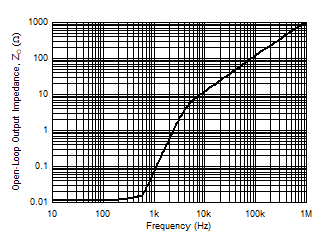Figure 23. Open-Loop Output Impedance vs FrequencyGain = 1, 10-mV step
Figure 25. Small-Signal Overshoot vs Capacitive Load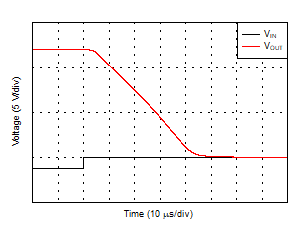Figure 27. Positive Overload Recovery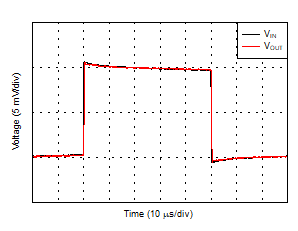Gain = 1, 10-mV step
Figure 29. Small-Signal Step Response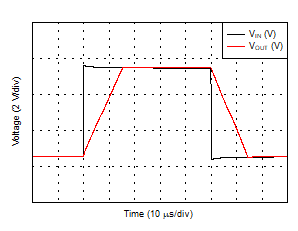Gain = 1, 10-V step
Figure 31. Large-Signal Step Response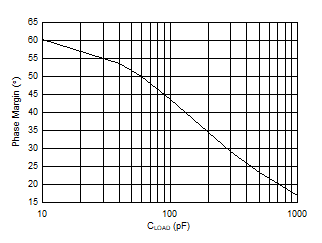Figure 33. Phase Margin vs Capacitive Load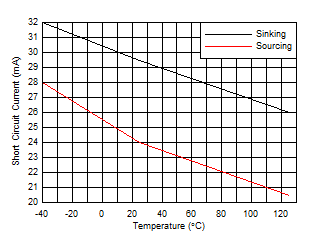Figure 35. Short Circuit Current vs Temperature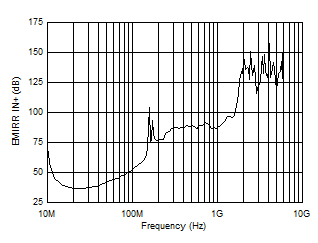Figure 37. EMIRR vs Frequency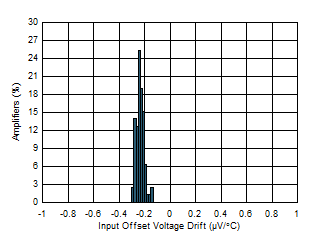Figure 2. Offset Voltage Drift (-40°C to 125C°C)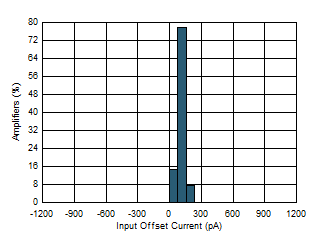Figure 4. Input Offset Current Distribution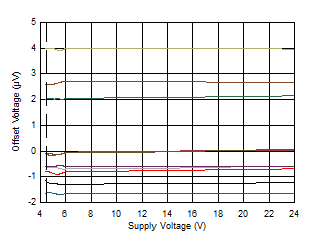Figure 6. Offset Voltage vs Supply Voltage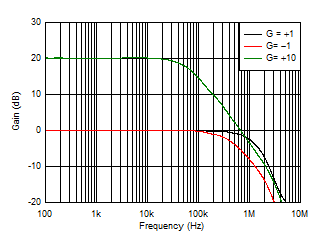Figure 8. Closed-Loop Gain vs Frequency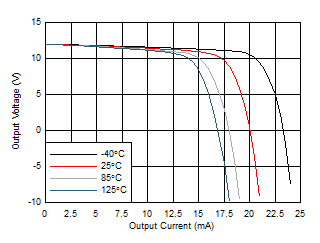Figure 10. Output Voltage Swing vs Output Current (Sourcing)Figure 12. CMRR and PSRR vs FrequencyFigure 14. PSRR vs Temperature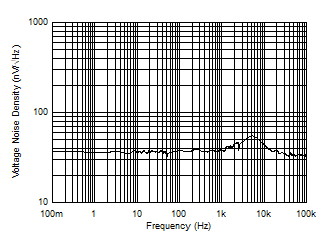Figure 16. Input Voltage Noise Spectral Density vs FrequencyFigure 18. THD+N vs Output Amplitude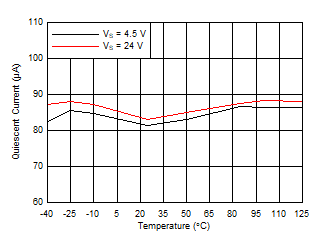Figure 20. Quiescent Current vs Temperature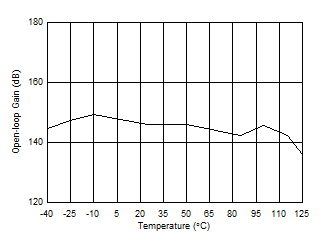RL = 2 kΩ
Figure 22. Open-Loop Gain vs Temperature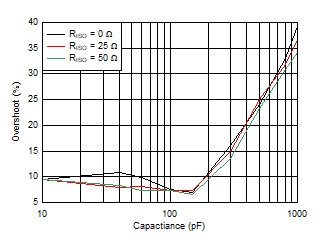Gain = –1, 10-mV step
Figure 24. Small-Signal Overshoot vs Capacitive Load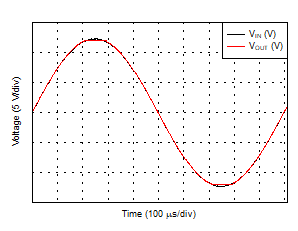Figure 26. No Phase ReversalFigure 28. Negative Overload Recovery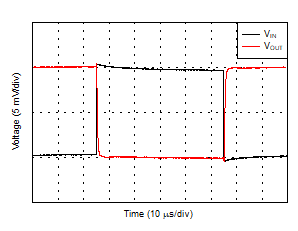Gain = –1, 10-mV step
Figure 30. Small-Signal Step ResponseGain = –1, 10-V step
Figure 32. Large-Signal Step Response1-V step, 0.1% settling
Figure 34. Settling Time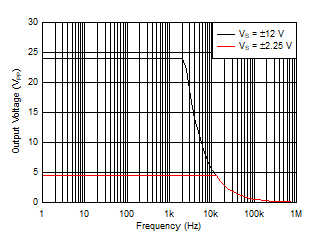Figure 36. Maximum Output Voltage vs Frequency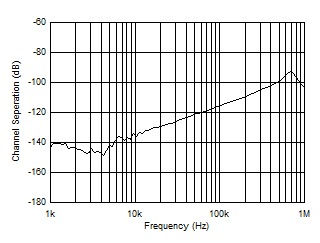Figure 38. Channel Separation